# Forster-Swan theorem

(diff) ← Older revision | Latest revision (diff) | Newer revision → (diff)

Swan–Forster theorem

An example of a local-global principle in commutative algebra (cf. also Local-global principles for large rings of algebraic integers; Local-global principles for the ring of algebraic integers). That is, it provides a method by which local information can be lifted to the whole ring or module. In the case of the Forster–Swan theorem the information consists of the minimal number of elements required to generate a given finitely-generated module. The theorem itself, and also the methods developed in proving it, have found applications in commutative algebra, algebraic geometry and algebraic-theory; see [a5] and [a6].

Letbe a commutative ring, and letbe a finitely-generated-module (cf. also Module). Suppose that one wants to compute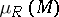, the minimal number of generators of. This can be a difficult task in general, but one can easily compute the number of generators oflocally. Thus, letbe a prime ideal of, and denote bythe quotient ring of. By the Nakayama lemma (cf. also Jacobson radical),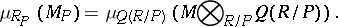(a1)

This number will be denoted by, and it will be called the local number of generators ofat. Note that the right-hand side of (a1) is equal to the dimension of a vector space, and as such is easily computed. Hence, an upper bound forin terms of the numbers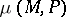, for the various prime idealsof, would be a very desirable result.

In order to have a means of guessing what upper bound one might expect, one turns to the equivalence between projective modules and fibre bundles. Letbe an-dimensional CW-complex and denote by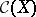its ring of continuous functions. Ifis a real vector bundle of dimensionover, then there exists a vector bundlesuch that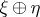is a trivial bundle. Moreover, one can assume thathas dimension smaller than or equal to; see [a4], Chapt. 8, Thms. 1.2, 1.5. Thus, the-module of sections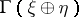is isomorphic to a free module of rank at most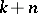. Sinceis a homomorphic image of this free module,elements must be sufficient to generate it.

Using the above as a guide, one may now return to the general algebraic setting. First, the rank of a projective module at a prime is equal to its minimal number of generators. Secondly, the role of the dimension of the topological space will be played, in the algebraic setting, by the Krull dimension of the base ring (cf. also Dimension). Finally, taking into account the fact that the local number of generators of a module need not be the same at every prime, the topological analogy suggests that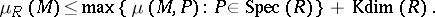Assuming thatis a commutative Noetherian ring, this is just what R.O. Forster proved in [a3]. Following a suggestion of J.-P. Serre, Forster's result was later generalized by R.G. Swan to rings whose maximal spectrum is Noetherian, [a9] (cf. also Spectrum of a ring). Denoting by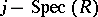the set of prime ideals that are intersections of maximal ideals, the Forster–Swan theorem can be stated as follows. Letbe a commutative ring and assume thatis a Noetherian space. Ifis a finitely-generated-module, then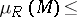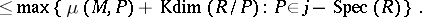A number of further improvements are possible. For instance, one can discard all-primes outside the support of, and the Krull dimension can be replaced by the-dimension. For details on these and other improvements, see [a2] or [a5], where a proof of the theorem is given based on the notion of a "basic element" . These methods also produce a similar bound for the stable rank of a module; see [a2], Corollary 6.

Given the importance of the Forster–Swan theorem, it was natural to ask whether it could be generalized to non-commutative rings. The obstacle was the usual problem of localizing at a prime in a non-commutative algebra. However, ifis a prime ideal of a Noetherian ring, thenis a prime Noetherian ring. Hence it must have a quotient ring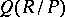by Goldie's theorem. Therefore, the right-hand side of (a1) makes sense, and there is still a good definition ofin this case. This led J.T. Stafford, building on earlier work of R.B. Warfield, to a proof of the Forster–Swan theorem for right and left Noetherian rings [a7]. A simpler proof, that also works for right Noetherian rings, can be found in [a1]. For applications of the Forster–Swan theorem to non-commutative algebra, see [a8].

How to Cite This Entry:
Forster-Swan theorem. Encyclopedia of Mathematics. URL: http://encyclopediaofmath.org/index.php?title=Forster-Swan_theorem&oldid=12186
This article was adapted from an original article by S.C. Coutinho (originator), which appeared in Encyclopedia of Mathematics - ISBN 1402006098. See original article